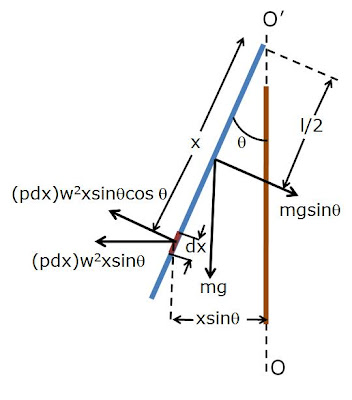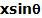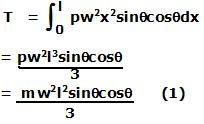Thursday, February 12, 2009

Irodov Problem 1.270Consider an infinitesimally small piece of the rod of length dx at a distance x from O'. If the linear density (mass per unit length) of the rod is p then the mass of this piece if pdx. The piece rotates about the axis OO' at a radius ofand so the centrifugal force acting on the rod (as seen in the rotating frame) is given by. The component of the centrifugal force that is perpendicular to the rod is responsible for inducing a torque on the rod and is given by,as shown in the figure. The moment arm of the force is x and so the torque acting on the piece of rod is given by,The net torque acting on the rod due to the centrifugal force is given by,The force of gravity mg acts at the CG (center) of the rod at a distance l/2 from O' and generates a counter-torque to the torque due to the centrifugal force. The component of gravity in the direction perpendicular to the rod is given byand thus the torque generated by gravity if given by. In order for the rod to rotate at a stable angle, the torque due to gravity must exactly counter-balance that due to the centrifugal force. Thus, we have,